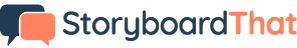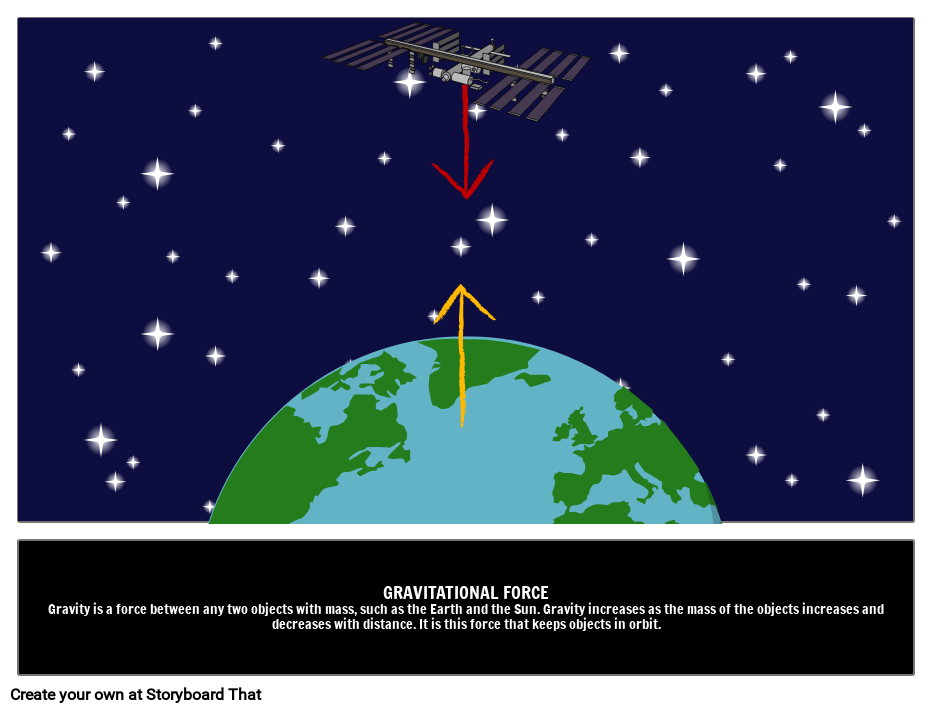x### Want to create storyboards like this one?### Try Storyboard That!

Create a storyboard

Gravity is a force between any two objects with mass, such as the Earth and the Sun. Gravity increases as the mass of the objects increases and decreases with distance. It is this force that keeps objects in orbit.Gravity is an attractive force between any two objects that have mass. The strength of the gravitational force depends on two factors: the amount of mass and the distance between the objects. It is one of the four fundamental forces, the other three being electromagnetic, strong, and weak forces.

Gravitational forces keep us and everything on Earth stuck the ground and gives objects weight. Gravitational field strength varies on different planets. This means that while objects may have the same mass on wherever they are in the solar system, their weight can vary. The strength of gravity on Earth is 9.8N/kg whilst on the moon the gravitational field strength is one sixth of that. Gravitational force also keeps every object from the international space station to planets in orbit. People often think that the reason that astronauts float on the international space station is due to a lack of gravity. The strength of gravity at the distance the space station is from Earth is 90% of the strength on Earth. The reason the astronauts float is that they are effectively in free fall around the Earth. The gravitational force also keeps groups of objects together, like stars in our galaxy or planets in our solar system.Galileo experimented by rolling balls down inclines and found that objects fall at the same increasing rate, proportional to how long they've been falling. Gravitational force was first described mathematically by British Scientist Isaac Newton. The famous story says that Newton discovered gravity whilst he was watching a falling apple. While the part of the story about the apple isn’t true, Newton did describe gravity as a force. This led to an equation that allowed the strength of gravity to be calculated mathematically using the masses of the objects and the distance between them.

The next large revolution in understanding gravity came with Einstein’s paper on general relativity. In this, he built on the ideas of Newton and described gravity as the warping of spacetime. Einstein predicted that this warping of space time would also cause light to bend around objects with a lot of mass. Recently, the existence of gravitational waves has been discovered. These waves were predicted over 100 years ago by Einstein and their discovery provides further support for Einstein’s theory.